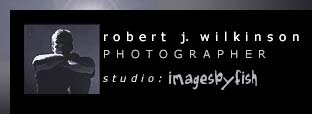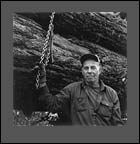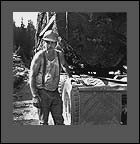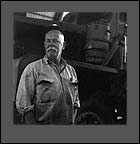P R O F I L EG A L L E R YO R D E RC O N T A C T    I N F OP  E  O  P  L  E    &    L  I  F  E  S  T  Y  L  E

"If I can tell the slightest truth or reveal the smallest insight
of a person, then . . ."

G A L L E R Y
F E A T U R E D   E X H I B I T
L A N D S C A P E
P E O P L E   &   L I F E S T Y L E  - 1
> P E O P L E   &   L I F E S T Y L E  - 2
H U M A N   S H A P E   &   F O R M
S T I L L   L I F E
E X P E R I M E N T A L" c h a r l e n e "" e d "" j o h n "" d a v e "" w a r r e n "" s t e v e " back to page one >  S E N D   T H I S   S I T E   T O   A   F R I E N DT O P© 2001 - 2005   R o b e r  t   J   W i l k i n s o n             P r i v a c y   P o l i c ys i t e   d e s i g n